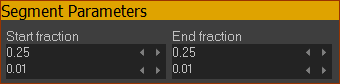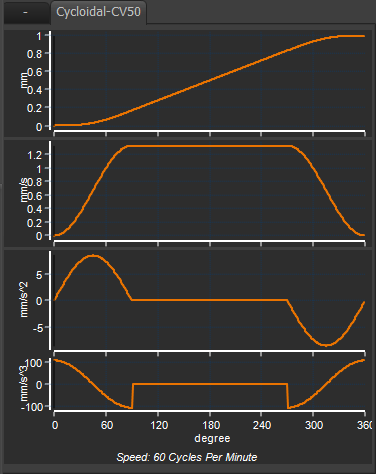﻿ MotionDesigner Reference & User Interface > Motion Laws > Cycloidal-CV50 Motion-Law

# Cycloidal-CV50 Motion-Law

Navigation:  MotionDesigner Reference & User Interface > Motion Laws >

# Cycloidal-CV50 Motion-Law

### Cycloidal-CV50 Cam-Law, Motion-Law

The Cycloidal-CV50 has three phases:

 Phase 1: Acceleration : first ½ wave of a Sine function : 25% of the Segment-Width. Phase 2: Zero Acceleration : Constant Velocity : 50% of the Segment-Width. Phase 3: Deceleration : last ½ wave of a Sine function : 25% of the Segment Width

 1.Select a Ramp Motion-Law 2.Open the Segment Editor 3.Edit the Segment Parameters Start-Fraction = 0.25, End-Fraction = 0.25

Segment Parameters - for the RAMP motion-lawStart-Fraction × 100 = 25% of Segment-Width End-Fraction × 100 = 25% of Segment-Width

Start-Fraction × 100 = 25% of Segment-Width

End-Fraction × 100 = 25% of Segment-Width

#### Segment EditorandBlend-Point Editor

You CAN specify the:

 •Start-Position The Start-Position usually flows from the End-Position of the •End-Position

You CANNOT specify the:

 •Start-Velocity, End-Velocity •Start-Acceleration, End-Acceleration •Start-Jerk, End-Jerk

Segment ParametersStart-Fraction × 100 = % of Segment-Width End-Fraction × 100 = % of Segment-Width

Segment-Range

 •Start-Range •End- Range 0 ≤ Start-Range < End-Range ≤ 1Cycloidal-CV50 (50% is Constant-Velocity (with Ramp Motion-Law / Cam-Law

Cycloidal-CV50

Symmetrical

Position, Velocity and Acceleration Continuity

Finite, but four Jerk discontinuities.

Motion-Law Coefficients

Velocity Coefficient: Cv = 1.33

Acceleration Coefficient: Ca= 8.378

Jerk Coefficient: Cj= ±105.276

Jerk at Cross-Over 0.25 Cross-over@0.25 q: Cj(co)= ±105.276 (Effectively)

#### Application Notes:

Dynamic Performance

This Motion-Law is recommended in applications where you need the peak velocity to be low when compared to others.

It has a large Peak Acceleration, with a short duration, thus more likely to induce vibrations.

Pressure Angle Considerations

It gives a relatively low pressure angle - because of its low peak velocity.

Drive Torques

This Motion-Law is not recommended in many applications as it will give a sudden change in torque. The input transmission rigidity would need to be high.